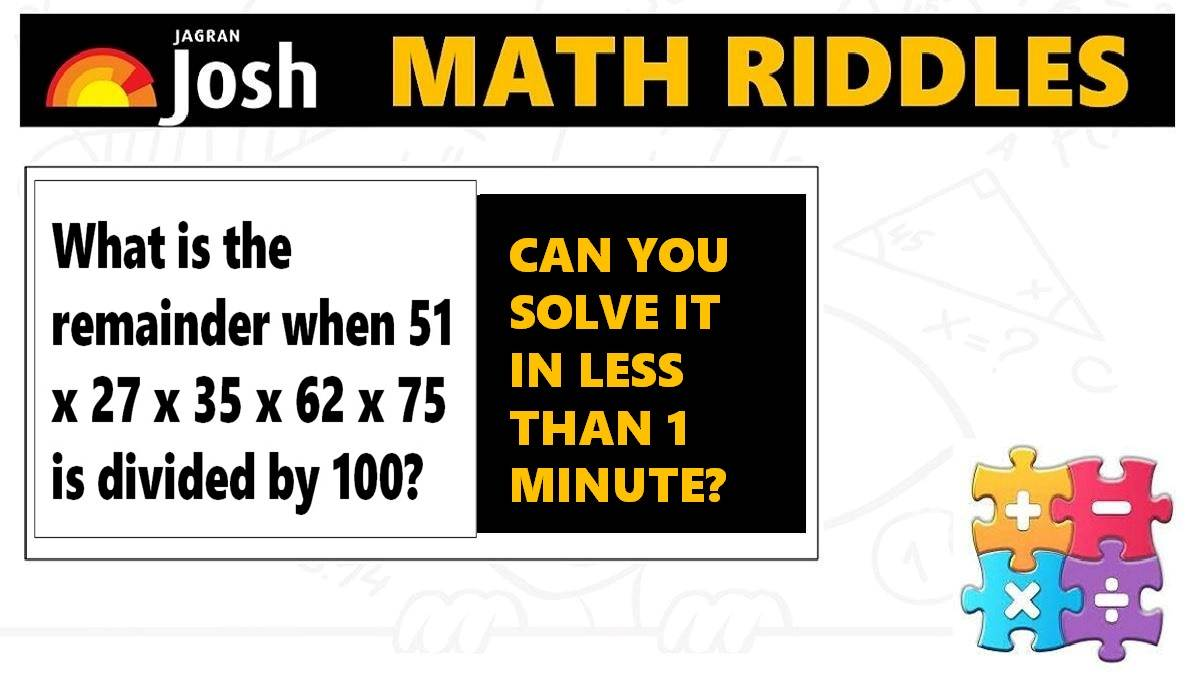# Math Riddles: Check Your Brain Power, Solve these Mathematics Puzzles

Math Riddles Challenge: Only a 100% genius can solve these mathematics puzzle in less than 1 minute each?Math Riddles: Check Your Brain Power, Solve these Mathematics Puzzles

Math Riddles: Only 1 out 5 people have been able to solve these tricky mathematics puzzles. Are you a genius who can find the right answer in less than 1 minute each? These mathematics puzzles are a preparation booster for the quantitative aptitude and reasoning ability sections in competitive government civil services exams, defence and banking exams.

## Solution

### Maths Logic Puzzle #1

Explanation:

In this mathematics puzzle, we shall multiply the digits in the ones place of each number.

1 x 7 x 5 x 2 x 5 = 350

We shall now divide 350 by 100, we shall get a remainder of 50.

Explanation:

## Tell us in comments: Did you solve these mathematics puzzles in less than 1 minute each?

Check out more math puzzles!

Also Read: Math Riddles: Can You Find The Next Number In These Math Puzzle Series?

Also Read: Math Riddles: Only 1 out 5 People Can Solve These 5-Minute Maths Puzzles

Also Read: Math Riddles: 5-Minute Maths Puzzles, Find the Answers in Less than 1 Minute

Get the latest General Knowledge and Current Affairs from all over India and world for all competitive exams.
खेलें हर किस्म के रोमांच से भरपूर गेम्स सिर्फ़ जागरण प्ले पर